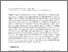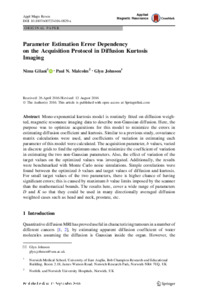# Parameter estimation error dependency on the acquisition protocol in diffusion Kurtosis imaging

Gilani, Nima, Malcolm, Paul N. and Johnson, Glyn (2016) Parameter estimation error dependency on the acquisition protocol in diffusion Kurtosis imaging. Applied Magnetic Resonance, 47 (11). 1229–1238. ISSN 0937-9347Preview PDF (Published manuscript) - Published Version Download (470kB) | Preview

## Abstract

Mono-exponential kurtosis model is routinely fitted on diffusion weighted, magnetic resonance imaging data to describe non-Gaussian diffusion. Here, the purpose was to optimize acquisitions for this model to minimize the errors in estimating diffusion coefficient and kurtosis. Similar to a previous study, covariance matrix calculations were used, and coefficients of variation in estimating each parameter of this model were calculated. The acquisition parameter, b values, varied in discrete grids to find the optimum ones that minimize the coefficient of variation in estimating the two non-Gaussian parameters. Also, the effect of variation of the target values on the optimized values was investigated. Additionally, the results were benchmarked with Monte Carlo noise simulations. Simple correlations were found between the optimized b values and target values of diffusion and kurtosis. For small target values of the two parameters, there is higher chance of having significant errors; this is caused by maximum b value limits imposed by the scanner than the mathematical bounds. The results here, cover a wide range of parameters D and K so that they could be used in many directionally averaged diffusion weighted cases such as head and neck, prostate, etc.View Item